# Rename mixed to fraction form

## Rename Mixed Numbers to Fraction Form with Circle Models

MIXED FORM TO FRACTION FORM
CORRECT:
ATTEMPTS:
SCORE:
PERCENT
NUMERATOR
DENOMINATOR
WHOLE OR MIXED FORM
FRACTION FORM

# RENAME MIXED TO FRACTION FORM INSTRUCTIONS

Follow the directions in the dialog box after pressing the <START> button. The <EXPLAIN> button may be pressed to see how to do the example.

Mixed Numbers to Fractions uses circle models to demonstrate how a number in mixed form can be renamed in fraction form.

The illustration below was made by Mixed to Fraction with Circles Designer. It shows the mixed number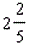. You are to writein fraction form, with only a numerator and denominator.

In this example, you will notice that each of the two whole circles has 5 colored pieces and the part circle has 2 colored pieces, giving 12 colored pieces in all for a numerator of 12. The denominator is 5 because each circle has 5 equal parts, giving a fraction of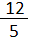Multiply the whole number 2 by the denominator 5 and then add the numerator 2 for the numerator 12 in the fraction form.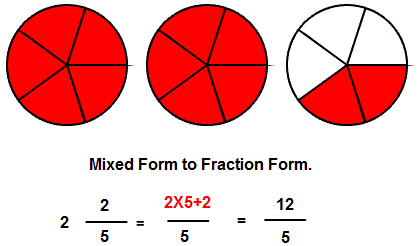Some textbooks call a fraction such as this improper, where the numerator is equal to or larger than the denominator. This is also known as the fraction form or a/b form of the number.

Another way to write the example is to think of each whole number as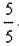. So in the above example you would have: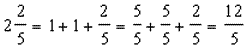For more instruction on renaming from mixed form to fraction form go to How to Rename from Mixed form to Fraction Form.

After you enter the fraction form answer you may press the <REPORT> button. The report will ask for your name but you may submit a code for your name. This report will give same results as on the dialog box. The report may be printed or e-mailed.